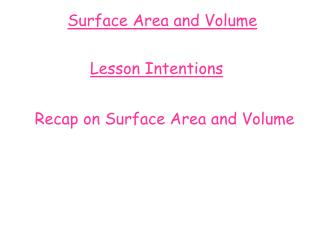DownloadDownload PresentationSurface Area and Volume

# Surface Area and Volume

Télécharger la présentation## Surface Area and Volume

- - - - - - - - - - - - - - - - - - - - - - - - - - - E N D - - - - - - - - - - - - - - - - - - - - - - - - - - -
##### Presentation Transcript

1. Surface Area and Volume Lesson Intentions Recap on Surface Area and Volume

2. Surface Area and Volume Which of the following units do we use to measure…. b) Volume a) Area ml cm3 mm2 cm2 cm m2 litres m3 km2 ** Always show your units at the end of your answer **

3. Surface Area and Volume Convert each weight to Kilograms : 2000g = 2Kg 7000g = 7Kg 12250g = 12.250Kg Convert each weight to grams : 9.3 Kg = 9300g 6.458Kg = 6458g 2.43Kg = 2430g Remember – Converting Kilograms to grams ……. …. Multiply by 1000 Remember – Converting grams to Kilograms ……. ….Divide by 1000

4. Surface Area and Volume Volume of a Cuboid To calculate the Volume of a cuboid, the formula we use is …… Length X Breadth X Height Volume = Height Breadth Length

5. Surface Area and Volume Volume of a Cuboid Calculate the volume of this Cuboid…….. 4cm 2cm 12cm

6. Surface Area and Volume Volume of a Cube To calculate the Volume of a cube, the formula we use is …… Length X Breadth X Height Volume = Remember … All sides have the same length on a cube!!! Height Breadth Length

7. Surface Area and Volume Volume of a Cuboid Calculate the volume of this Cube…….. 6cm

8. Surface Area and Volume Surface Area What is Surface Area?? ….. The total area of all the sides

9. Surface Area and Volume How many sides do each of the following Shapes have?........... 3 6 5

10. Surface Area and Volume Surface Area of A Cuboid To calculate the surface area of any cuboid the formula we use is………. Surface Area = Area of Front + Area of Back + Area of Top + Area of Bottom + Area of Side + Area of Side OR Surface Area = 2 x (Area of Front + Area of Side + Area of Top)

11. Surface Area and Volume Surface Area of a Cuboid Calculate the surface area of the following Cuboid…….. 4mm 5mm 10mm

12. Surface Area and Volume Volume of a Prism What is a prism?......... A prism is an object that when cut has the same cross section throughout

13. Surface Area and Volume Volume of a Prism To calculate the volume of any prism the formula is……… Volume = Area x Height …..and height is the height of the prism Where area is the area of the face of the prism….

14. Surface Area and Volume Volume of a Prism Calculate the area of the following Prism 25cm2 10cm

15. Surface Area and Volume Volume of a Triangular Prism We often have to work out the area of a cross-section before we can calculate the volume…. Calculate the area of the face Step 1. 1 breadth x height of triangle Area of Triangle = 2 Height of triangle Step 2. Calculate the volume of prism Area x Height of Prism Volume = Breadth of triangle Height of Prism

16. Surface Area and Volume Volume of a Triangular Prism 3cm 10cm 2cm Calculate the volume of this Prism

17. Surface Area and Volume Surface Area of a Triangular Prism A Triangular prism has how many faces?....... 5 A Triangular Prism has two identical triangles and 3 rectangles The formula to calculate the surface area of a triangular prism is........ Surface Area = (2 x area of triangle) + areas of 3 rectangular faces

18. Surface Area and Volume Surface Area of a Triangular Prism Calculate the surface area of the triangular prism..... 5cm 10cm 6cm

19. Surface Area and Volume Volume of a Cylinder A Cylinder is a circular prism, therefore its volume can be found by using the same formula for all prisms....... Volume = Area x height Since the cross section is a circle the formula reads ....... radius Volume = Π r2 x height r

20. Surface Area and Volume Volume of a Cylinder Calculate the volume of this cylinder........ 8cm 5cm

21. Surface Area and Volume Surface Area of a Cylinder A cylinder is made up of 2 basic shapes......... ....Rectangle .....Circle......

22. Surface Area and Volume Surface Area of a Cylinder To calculate the surface area of a cylinder we need to calculate 2 parts.... radius Area of top and bottom circles = 2 x π x r2 + Area of rectangle = 2 x π x r x height radius Total Surface area = 2πr2 + 2πrh

23. Surface Area and Volume Surface Area of a Cylinder Calculate the total surface area of this cylinder.......... 8cm 5cm